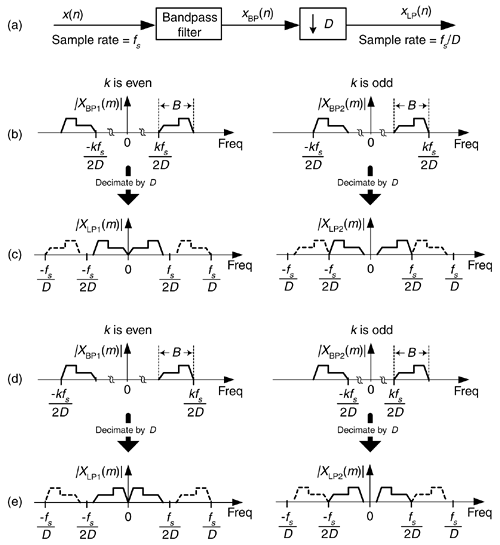# FREQUENCY TRANSLATION USING DECIMATION

We can frequency translate a real bandpass signal toward zero Hz, converting it to a lowpass signal, without the need for mixing multipliers using decimation by an integer factor D as shown in Figure 13-75(a). If the bandpass filter provides an output signal of bandwidth B Hz, located as shown in Figure 13-75(b) and Figure 13-75(d) where k is a positive integer, decimation by D will yield lowpass signals whose spectra are shown in Figure 13-75(c) and Figure 13-75(e) depending on whether integer k is odd or even. Please notice the inverted spectra in Figure 13-75(e). To avoid decimated output aliasing errors, we must satisfy the Nyquist criterion and ensure that xBP(n)'s bandwidth B is not greater than fs/2D.

Figure 13-75. Real bandpass signal translation using decimation by D.AmazonPrev don't be afraid of buying books Next